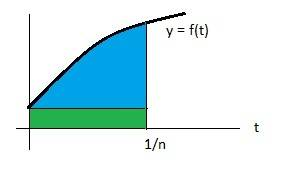# Integration question

• electronic engineer

#### electronic engineer

Hello,

I passed by this integration and couldn't understand the moving from the left hand to the right hand side.

$$\int_{0}^{1/n}f(t)dt=\frac{1}{n}f(0)$$

could you please tell me why this is??

Last edited:

the equation was actually
$$\lim_{n \to \infty }n\int_{0}^{1/n}f(t)dt= \lim_{n \to \infty }n\frac{1}{n}f(0)=f(0)$$

Hello,

I passed by this integration and couldn't understand the moving from the left hand to the right hand side.

$$\int_{0}^{1/n}f(t)dt=\frac{1}{n}f(0)$$

could you please tell me why this is??
I believe this is an approximation rather than an exact equality, although the approximation should get better with larger n.

What they are doing is approximating the integral on the left side by a rectangle whose width is 1/n and whose height is f(0). In the drawing below, the area of the green region is f(0) * (1/n). The value of the integral is the sum of the blue and green regions. The closer 1/n is to zero, the closer f(1/n) is to f(0), and the closer the approximation is, assuming that f is reasonably "well behaved."Last edited:
the equation was actually
$$\lim_{n \to \infty }n\int_{0}^{1/n}f(t)dt= \lim_{n \to \infty }n\frac{1}{n}f(0)=f(0)$$
You can actually prove this without making approximations: Let ##F(x)## be an antiderivative of ##f(x)##. Then
$$\lim_{n\rightarrow\infty}n\int_0^{\frac{1}{n}}f(t)\mathrm d t = \lim_{n\rightarrow\infty} \frac{F(\frac{1}{n})-F(0)}{\frac{1}{n}} = F^\prime(0) = f(0) \text{.}$$

Dear Mark44 and rubi,

thank you both for providing the information. It was very helpful. Thank you very much indeed! :-)